# RS Aggarwal Solutions For Class 7 Maths Exercise 1C Chapter 1 Integers

The pdf of RS Aggarwal Solutions for the Exercise 1C of Class 7 Maths Chapter 1, Integers are available here. To cover the entire syllabus in Maths, the RS Aggarwal is a necessary material as it gives a wide range of questions that test the students understanding of concepts. Our specialist staff have solved the problems step by step with neat descriptions. Students who aspire to score good marks in Maths, then refer RS Aggarwal Solutions for Class 7.

## Download the PDF of RS Aggarwal Solutions For Class 7 Maths Chapter 1 Integers – Exercise 1C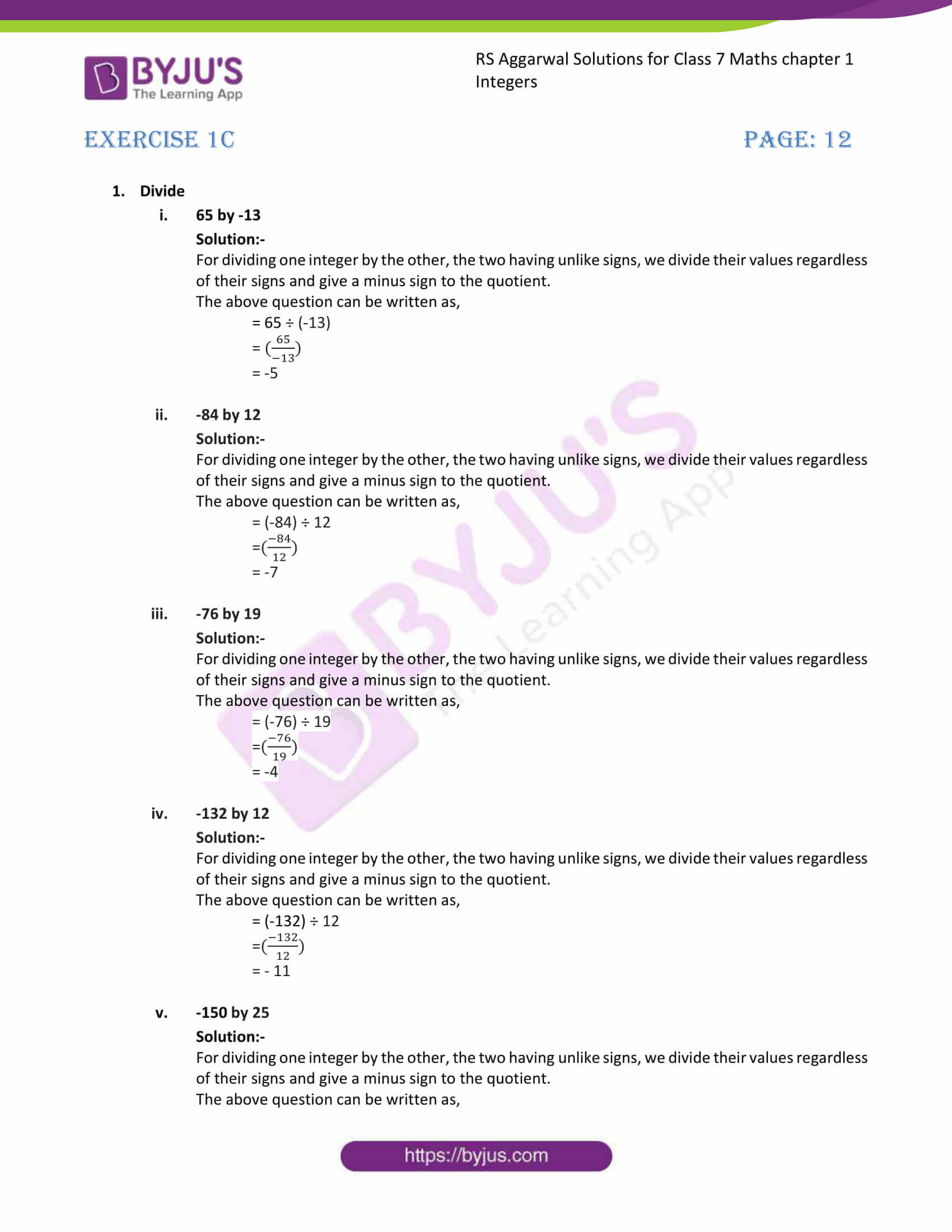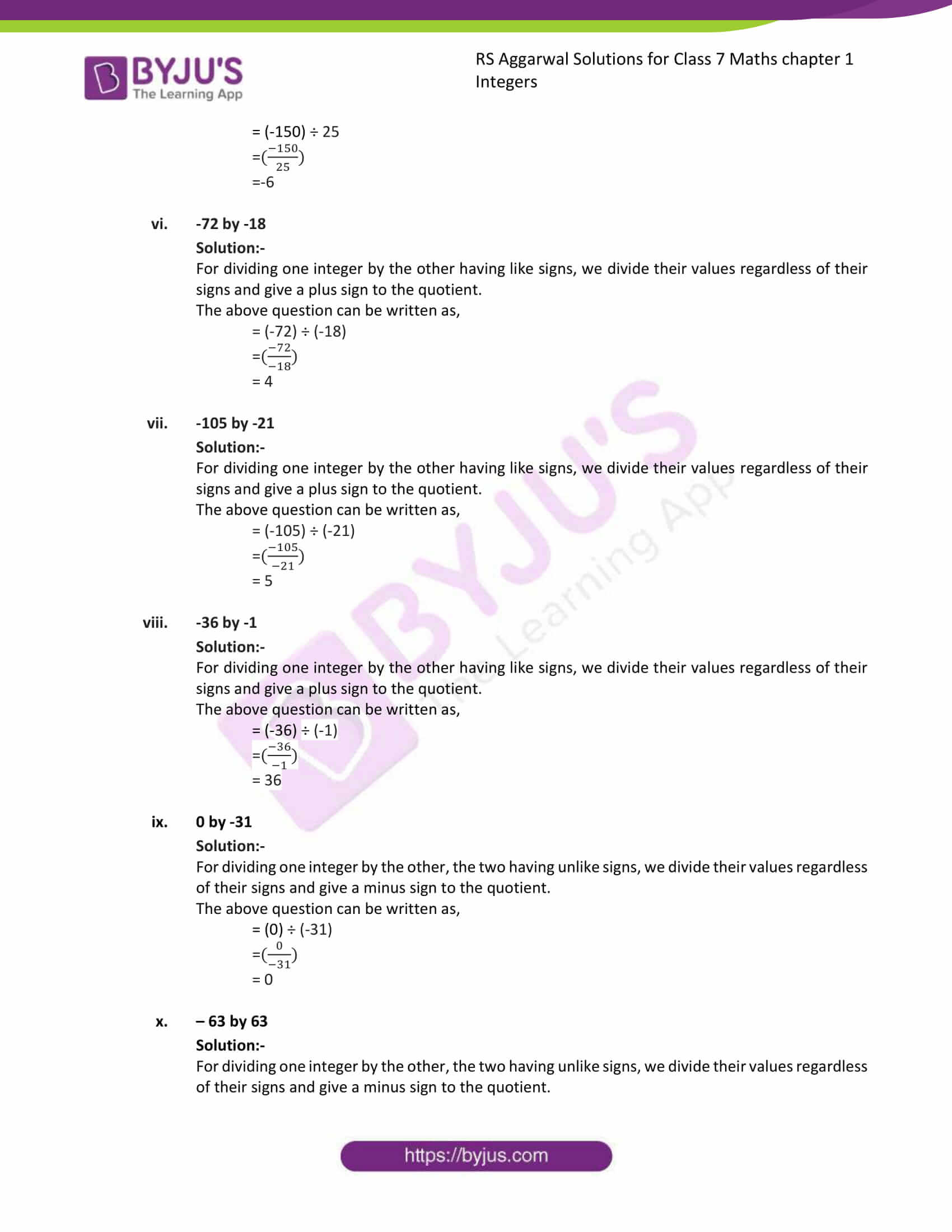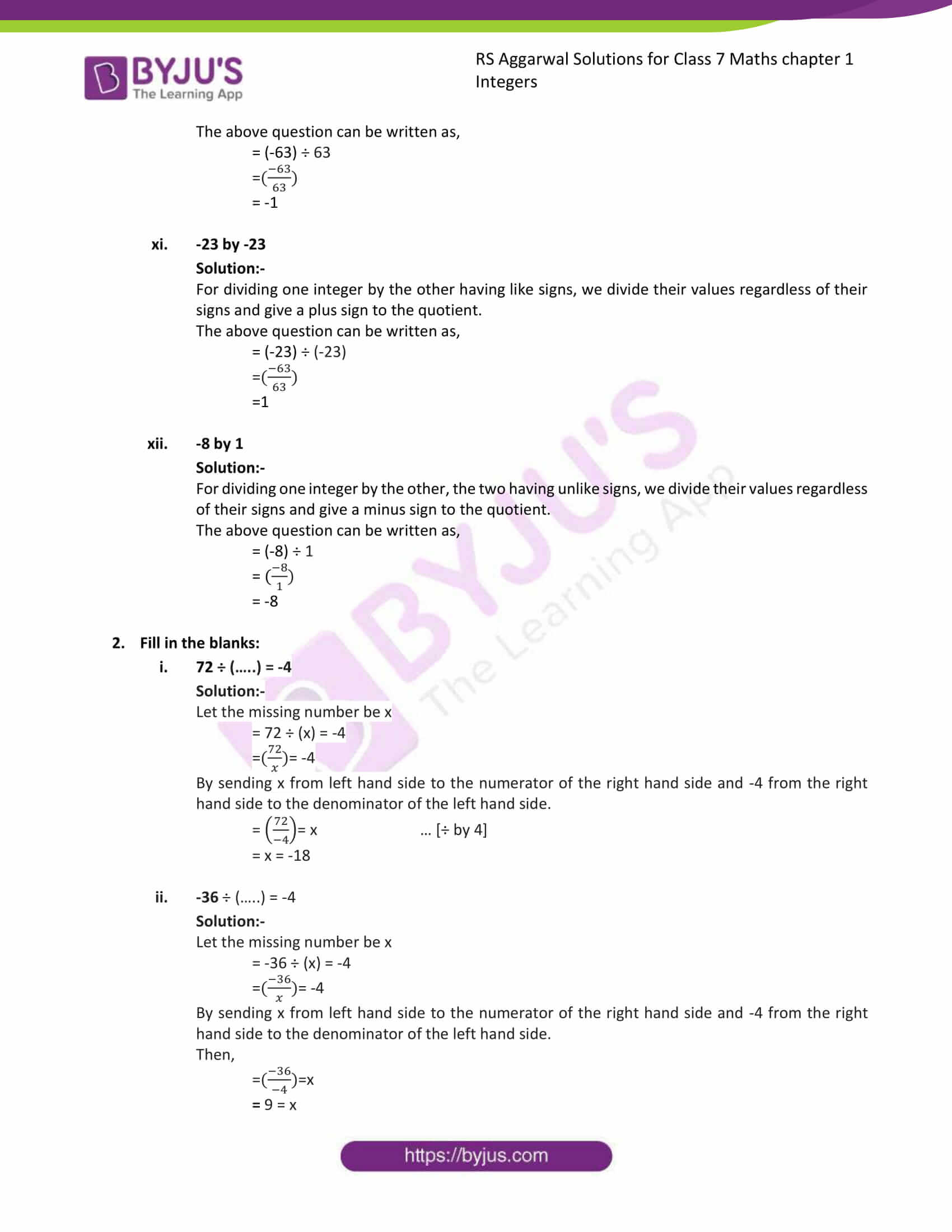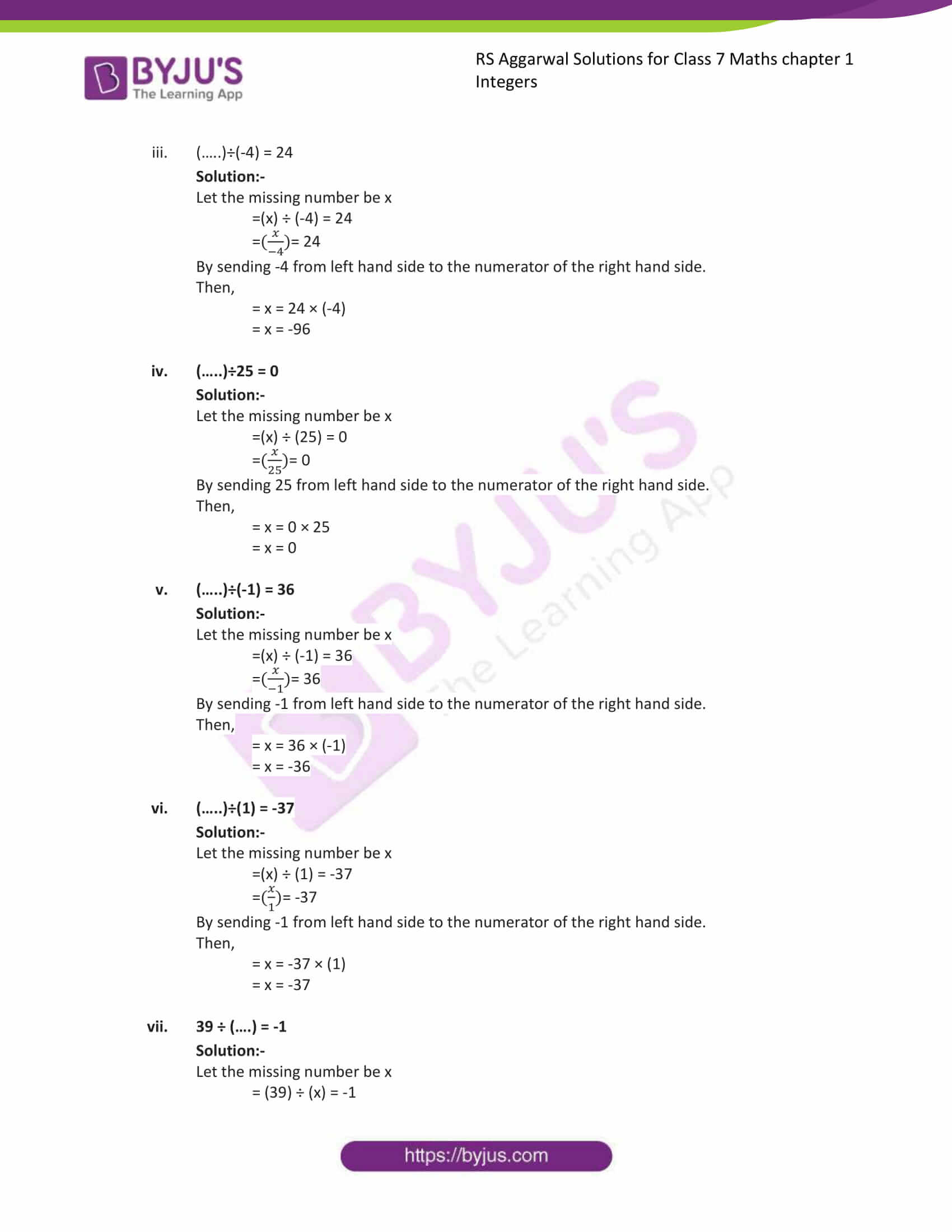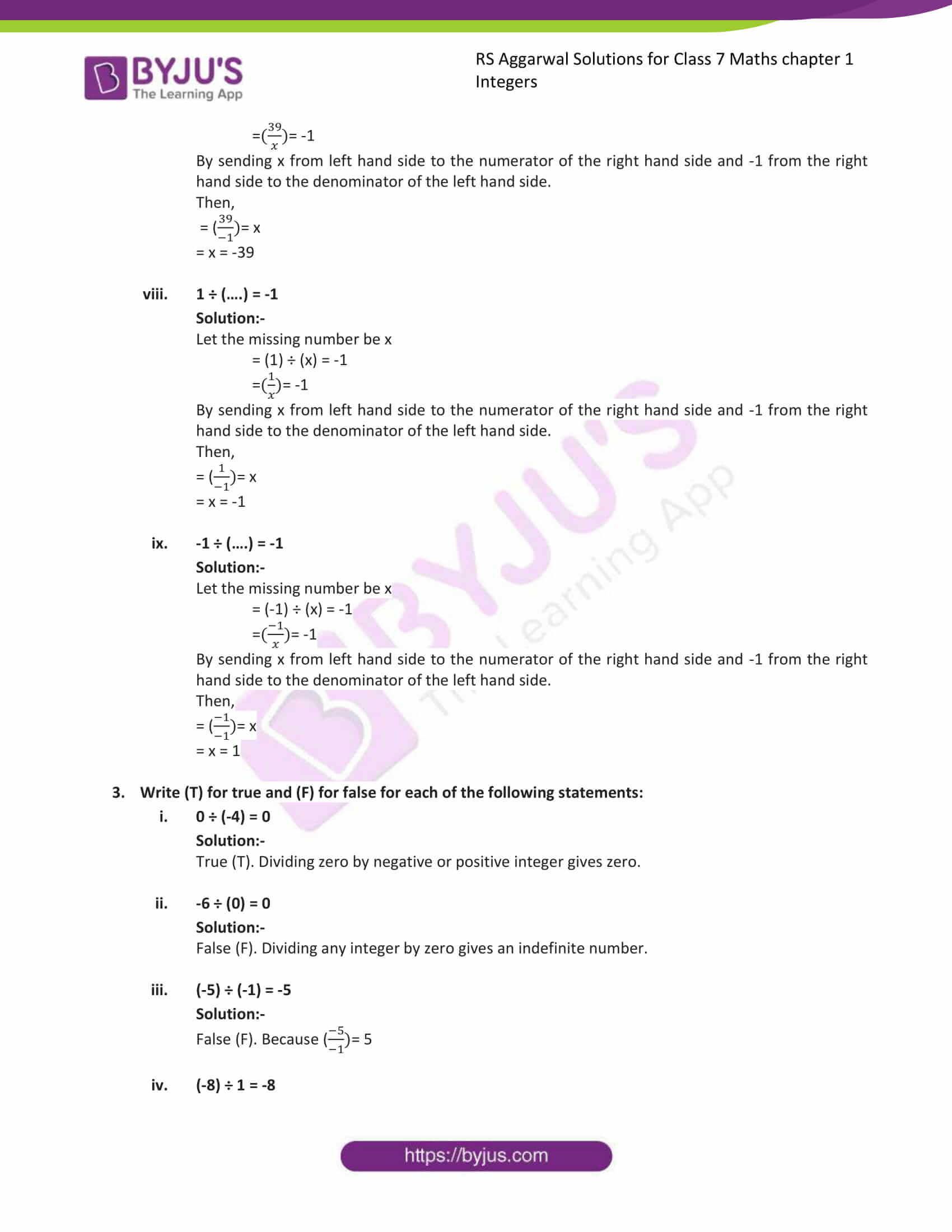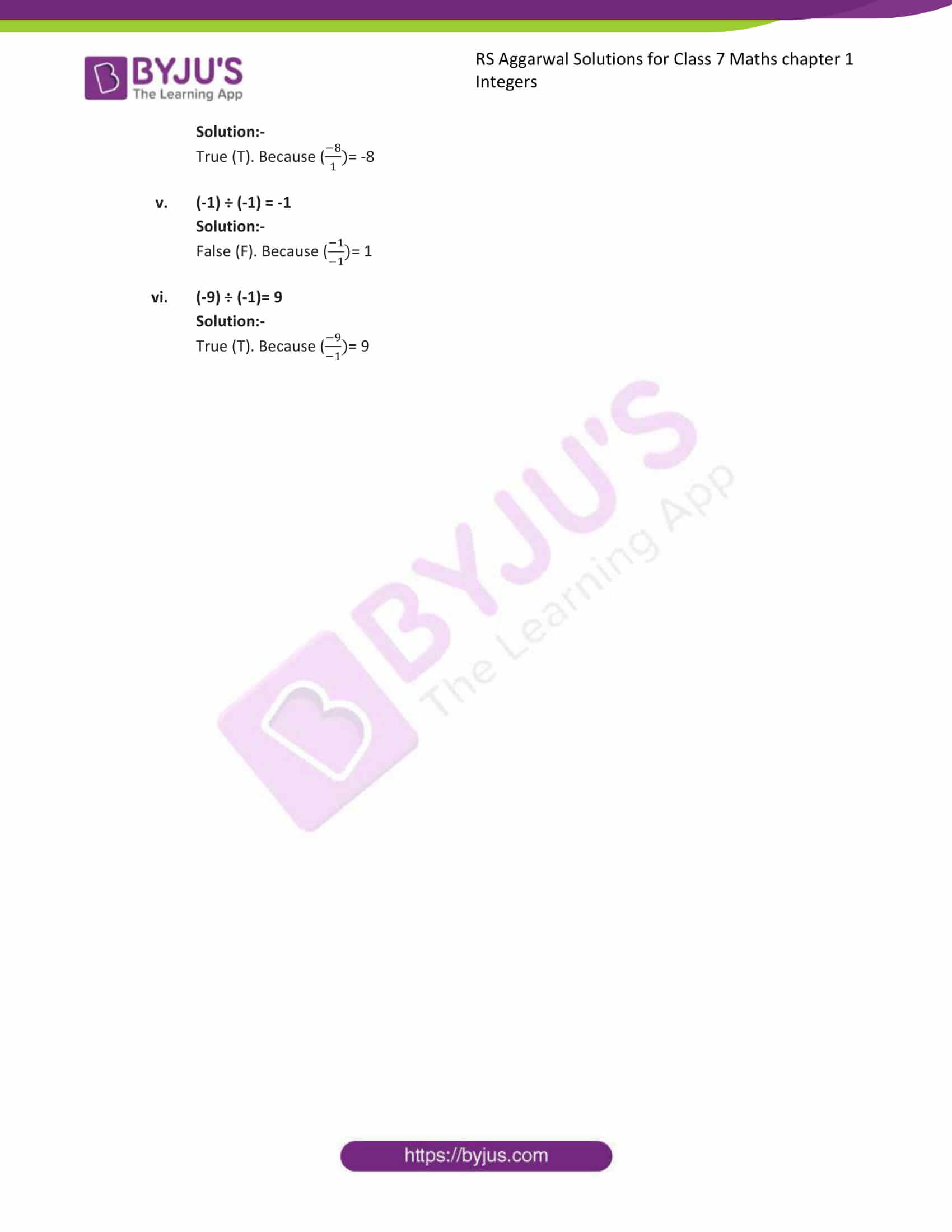### Access answers to Maths RS Aggarwal Solutions for Class 7 Chapter 1 – Integers Exercise 1C

1. Divide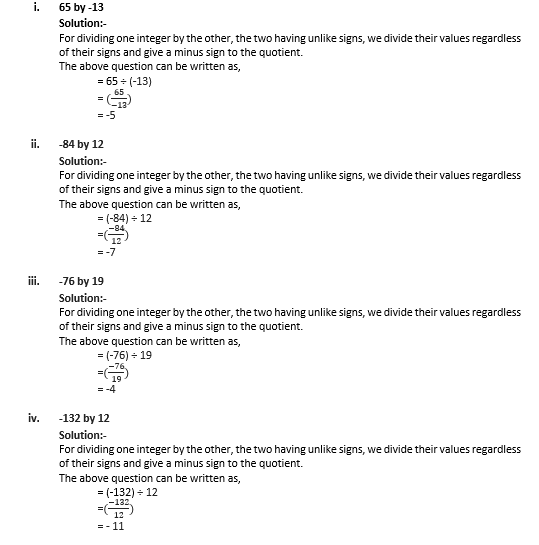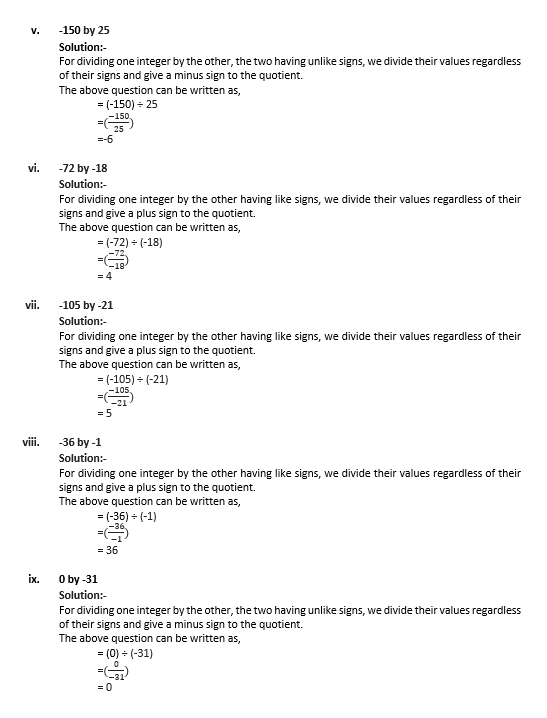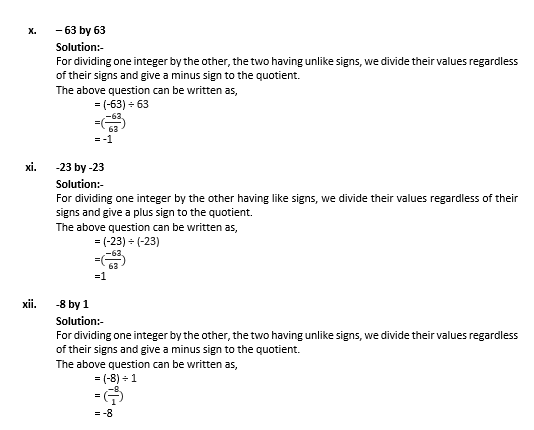2. Fill in the blanks: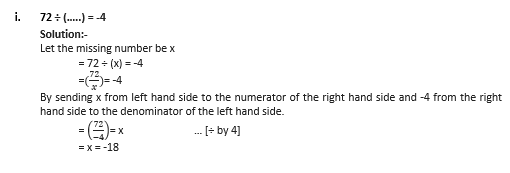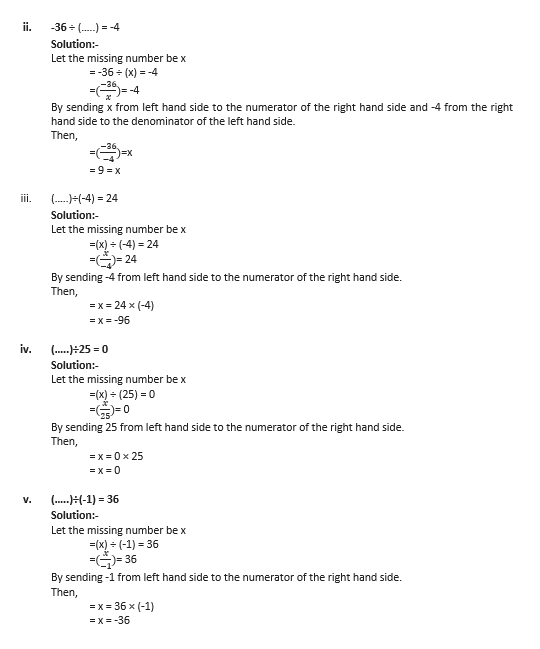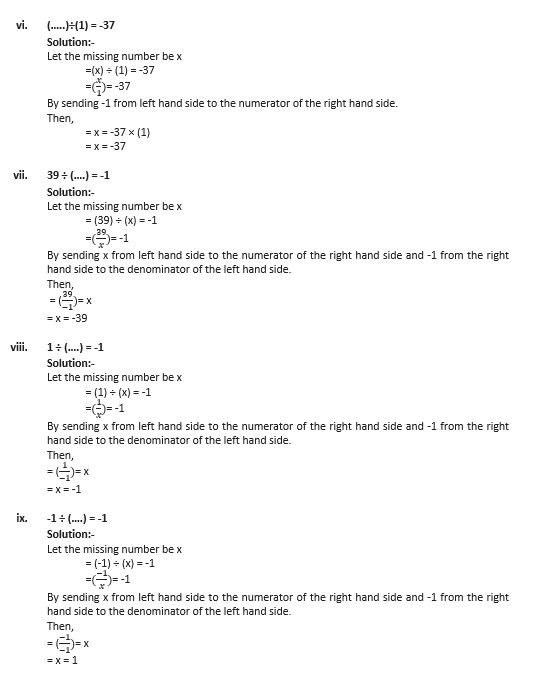3. Write (T) for true and (F) for false for each of the following statements: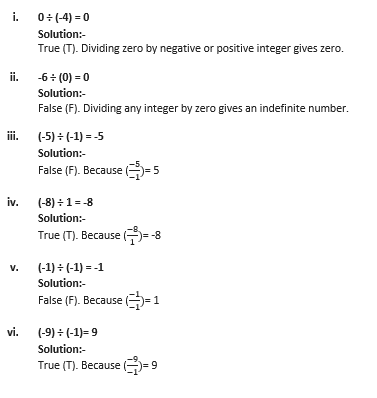### RS Aggarwal Solutions for Class 7 Maths Exercise 1C Chapter – 1 Integers

RS Aggarwal Solutions For Class 7 Maths Chapter 1 Integers Exercise 1C has problems which are based on the division of Integers and properties of division of integers. Students are suggested to try solving the questions from RS Aggarwal Solutions book of Class 7 and then refer to these solutions to know the best way of approaching the different questions.